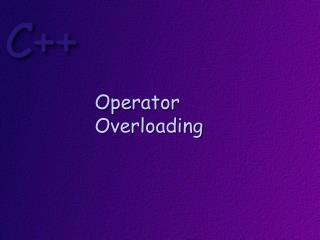DownloadDownload PresentationDownload Presentation- - - - - - - - - - - - - - - - - - - - - - - - - - - E N D - - - - - - - - - - - - - - - - - - - - - - - - - - -
##### Presentation Transcript

2. Objectives At the conclusion of this lesson, students should be able to Explain what operator overloading is Write code that overloads the standard binary operators Explain the rules for operator overloading

3. The expression a + b * c is simpler to understand then the expression plus(a, times(b, c) )

4. Therefore, in C++ you can define new meanings for the C++ operators that operate on objects derived from classes that you have defined.

5. Some Rules: An operator is overloaded by writing a function whose name is the word operator, followed by the symbol for the operator being overloaded. With some exceptions, these operator can be written as member functions or as non-member functions. You cannot change the meaning of any of the C++ operators for built in data types. For example, you cannot change how plus works on integer data. Precedence and associativity are fixed by the language. You cannot change these for an operator.

7. Class Number { public: Number( ); Number(int); intgetValue( ); private: inttheValue; };

9. Note that when you write a = b + c; the values of b and c do not change.

10. When using a member function we will use the following terminology (a, b, and c are objects of the Number class): this is the right hand operand (rho) a = b + c; This is the left hand operand (lho)

11. Overloading a binary operator as a member function Number Number::operator+(const Number& rho) { intnewValue = theValue + rho.getValue( ); Number newNumber(newValue); return newNumber; }

12. Question: Why is this Number object not being returned by reference? Number Number::operator+(const Number& rho) { intnewValue = theValue + rho.getValue( ); Number newNumber(newValue); return newNumber; }

13. Now, when the compiler sees the statement a = b + c; it generates the code a = b.operator+(c); the message is sent to the left hand operand the right hand operand is passed as the parameter.

14. Overloading a binary operator as a non-member function Number operator-(const Number& lho, const Number& rho) { intnewValue = lho.getValue( ) - rho.getValue( ); Number newNumber(newValue); return newNumber; }

16. ostream& operator<<(ostream& out, const Number& rho) { out << rho.getValue( ); return out; }